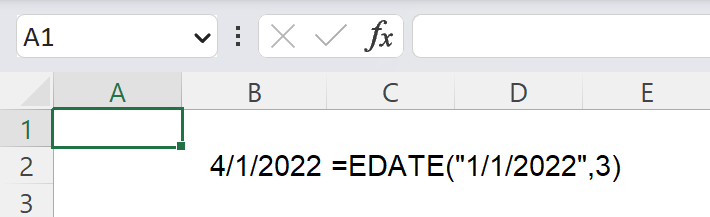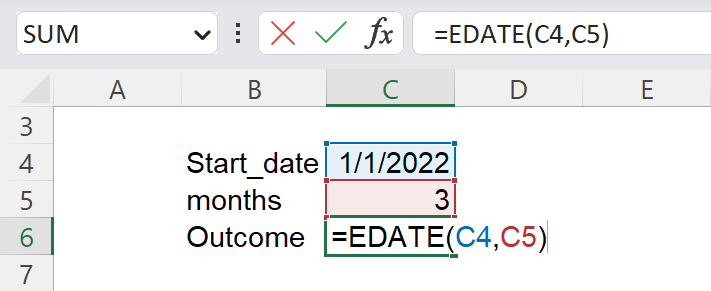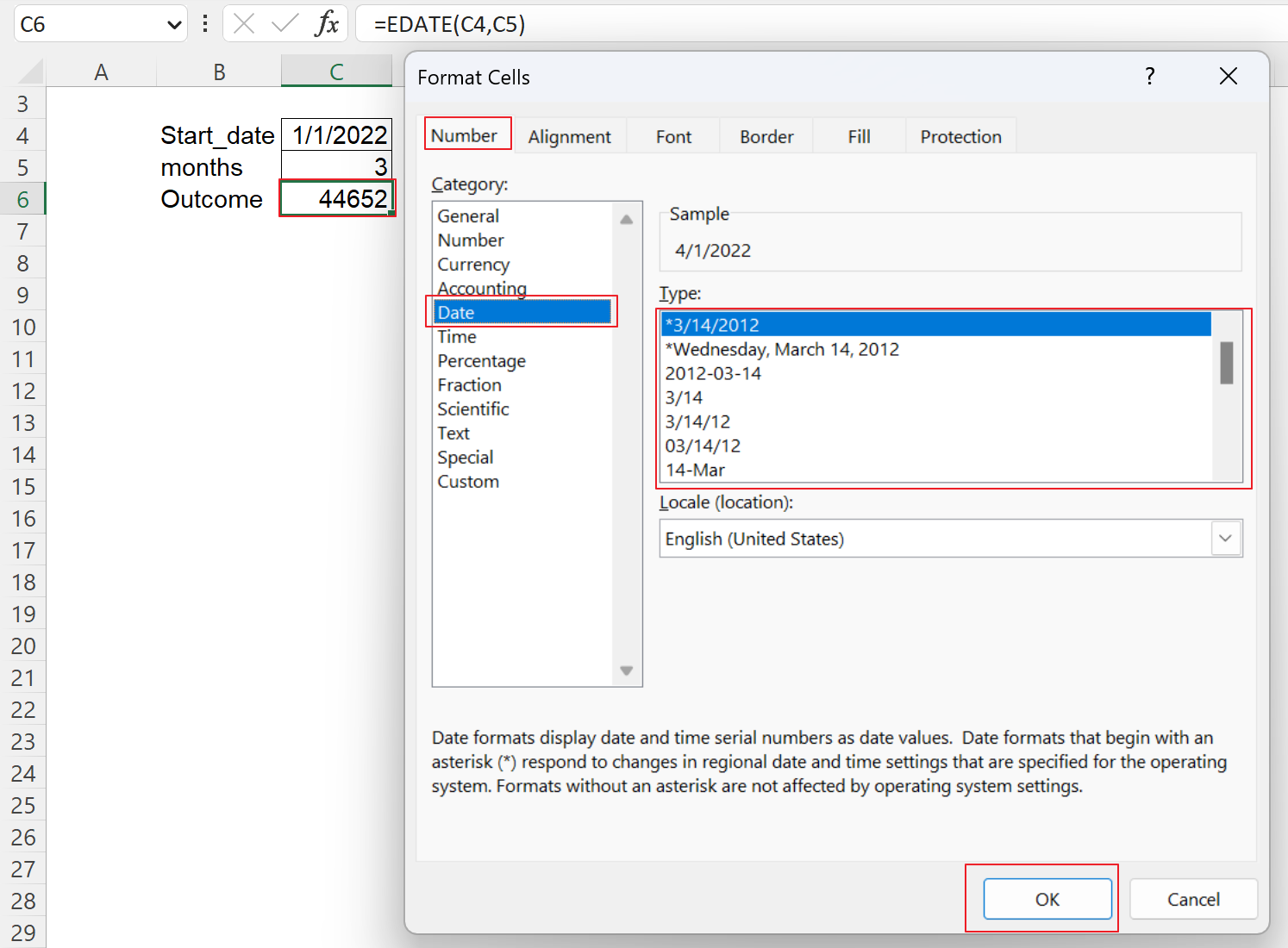Excel Formulas

# EDATE Function in Excel: Explained

In this article, you will learn how to use the EDATE formula in Excel.

## What is the EDATE formula in Excel?

The EDATE function in Excel returns the date (or its serial number) that is a specified number of months before or after a given date.

## When is the EDATE function useful in Excel?

The EDATE function in Excel can be helpful in various situations where you need to calculate future or past dates based on a given date and the number of months.

1. One use case is financial analysis, where you may need to calculate future or past due dates for loans, mortgages, or other financial instruments.
2. Another common use case is in project management, where you may need to calculate due dates for tasks or milestones based on the start date of a project.

## How to use the EDATE formula in Excel

The syntax for the EDATE function is as follows:

``=EDATE(start_date, months)``

The “start_date” argument is the date you want to base the calculation on, and the “months” argument is the number of months before or after the start date that you want to find. You can enter a positive number to get the number of months after the starting date and a negative number to get the number of months before the starting date. Both arguments, “start_date” and “months” are mandatory.

Note: In Excel, serial number 1 is given a date of January 1, 1900. The formula may return an error if the input starting date is invalid, especially when it is a text value. If the “months” argument is not an integer, it is truncated. Also, you need to enclose the date with quotation marks if you input it directly into the “start_date”. You can use the DATE function to ensure the “start_date” is a date format.

For example, if the start date is January 1, 2021, and you want to find the date three months after that, you would use the formula:

``=EDATE("1/1/2021", 3)``

This formula would return “April 1, 2021”.How to use the EDATE function by manual input in Excel

You can use cell references when you insert the EDATE formula, as shown below, assuming cell C4 contains a particular date and cell C5 includes an integer.

``=EDATE(C4,C5)``

This function returns 4/1/2022 or 44652, the serial number assigned to April 1, 2022.How to use the EDATE function by cell reference in Excel

## How to convert a serial number to a date

If the EDATE formula returns a serial number and you want to change it to a date format, follow the steps below.

1. Press “Ctrl” + “1” (for Windows) or “Cmd”+”1” (for Mac OS), which brings up the format cell dialog box.
2. Navigate to the “Date” category in the “Number” tab.
3. Select a date format you like from the list.
4. Click the “OK” button at the bottom right of the pop-up dialog box.How to convert a serial number to a date format in Excel

## Analyze your live financial data in a snap in Google Sheets

Are you learning this formula to visualize financial data, build a financial model, or conduct financial analysis? In that case, LiveFlow may help you automate manual workflows, update numbers in real-time, and save time. You can access various financial templates on our website, from the simple Income Statement to Multi-Currency Consolidated Financial Statement. Are you interested in this product but are an Excel user? That’s not a problem at all. You can connect Google Sheets to Excel quickly.